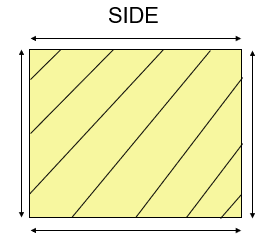# Area of Square

Area of Square is equal to (length of its side x length of side).

Area of Square = ( side x side )  = (side)²Question 1

Find the area of a square plot of side 8 cm?

Explanation

Sides of square = 8cm

Area of a square = ( side x side ) cm²

= ( 8 x 8 ) cm²

= 64 cm²

Hence, the area of a square plot is 64 cm²

Question 2

Find the area of a square plot of side 17 cm?

Explanation

Sides of square = 17cm

Area of a square = ( side x side ) cm²

= ( 17 x 17 ) cm²

= 289 cm²

Hence, the area of a square plot is 289 cm²

Question 3

Find the area of a square plot of side 14 cm

Explanation

Sides of square = 14cm

Area of square = ( side x side ) cm²

= ( 14 x 14 ) cm²

= 196 cm²

Hence, the area of square plot is 196 cm²

Question 4

Find the area of a square plot of side 25 cm

Explanation

Sides of square = 25cm

Area of square = ( side x side ) cm²

= ( 25 x 25 ) cm²

= 625 cm²

Hence, the area of square plot is 625 cm²

Question 5

Find the area of a square plot of side 6.5 cm

Explanation

Sides of square = 6.5cm

Area of square = ( side x side ) cm²

= ( 6.5 x 6.5 ) cm²

= 42.25 cm²

Hence, the area of square plot is 42.25 cm²